# Cantilever Thin Square PlateThe purpose of this test is to check the distorted meshes. You will use 2D meshes formed of parabolic quadrangle elements (QD8).Reference:

NAFEMS-Glasgow, Benchmark newsletter, April 1989, p.19.

##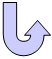Specifications

### Geometry Specifications

 Length: L = 10 m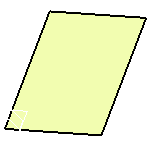Width: W = 10 m Thickness: th = 0.05 m

### Analysis Specifications

 Young Modulus (material): E = 200 GPa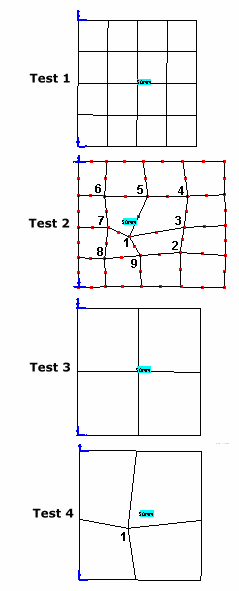Poisson's Ratio (material): ν = 0.3 Mesh Specifications: Parabolic quadrangle elements (QD8)    Constrained points For tests 1 and 3: regular For tests 2 and 4: Point 1: x = 4.0 and y = 4.0 Point 2: x = 2.25 and y = 2.25 Point 3: x = 4.75 and y = 2.5 Point 4: x = 7.25 and y = 2.75 Point 5: x = 7.5 and y = 4.75 Point 6: x = 7.75 and y = 7.25 Point 7: x = 5.25 and y = 7.25 Point 8: x = 2.25 and y = 7.25 Point 9: x = 2.5 and y = 4.75 Restraints (User-defined): Along y axis, Tx = Ty = Tz =  Ry = 0

##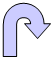Results

The results are given in the table below.
Normalized results (computed results divided by the reference solution) are listed.

 Mode Reference Test 1 Test 2 Test 3 Test 4 Computed  results [Hz] Normalized  results Computed  results [Hz] Normalized  results Computed  results [Hz] Normalized  results Computed  results [Hz] Normalized  results 1 0.421 0.419 0.995 0.420 0.998 0.419 0.995 0.420 0.998 2 1.029 1.023 0.994 1.032 1.003 1.024 0.995 1.028 0.999 3 2.582 2.576 0.998 2.606 1.009 2.697 1.045 2.703 1.047 4 3.306 3.295 0.997 3.317 1.003 3.420 1.034 3.540 1.071 5 3.753 3.733 0.995 3.930 1.047 3.900 1.039 3.993 1.064 6 6.555 6.841 1.044 6.799 1.037 6.677 1.019 6.844 1.044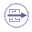To Perform the Test:

The cantilever_thin_square_plate_test2.CATAnalysis document presents a complete analysis of the test 2, computed with an irregular mesh formed of parabolic quadrangle elements (QD8).

To compute the three other tests, with other nodes of distribution, proceed as follow:

1. Open the CATAnalysis document.

2. In the Advanced Meshing Tools workbench, change the mesh specifications as indicated above.

3. Compute the case in the Generative Structural Analysis workbench.